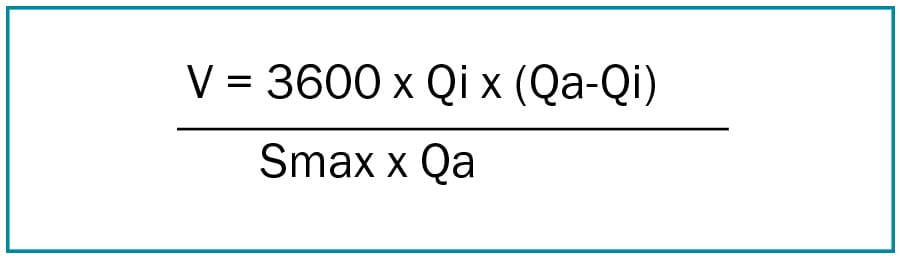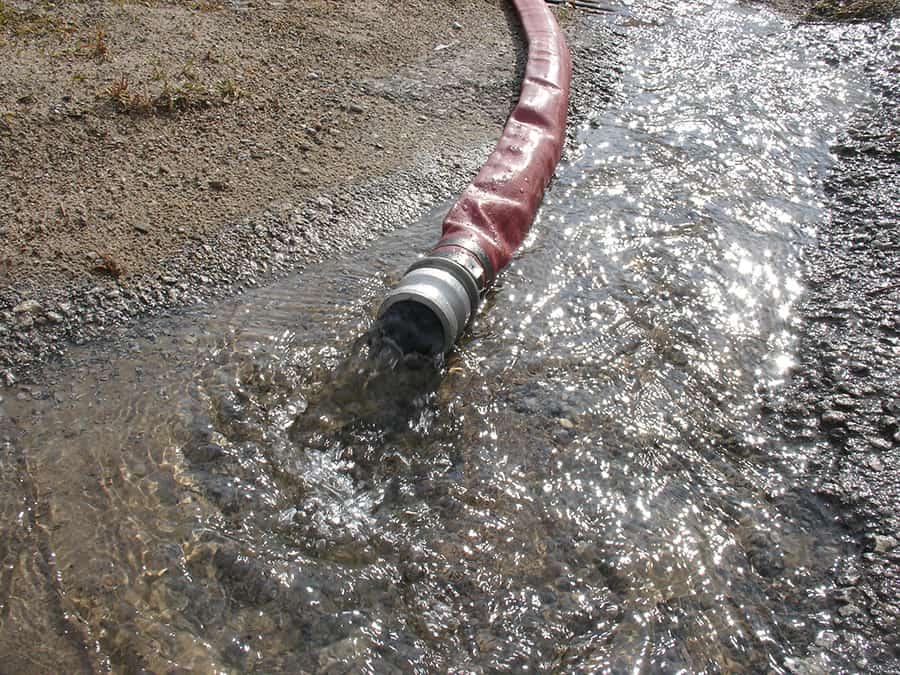Question: How is the volume of pump sumps or pits determined? Is there an optimum size?

Pumps in pits would normally be started and stopped by means of level switches of some type. There will be a maximum and minimum level in the pit. The volume between these levels is called the useful volume. The flow from the pump will change as the liquid level in the pit changes as the system resistance changes due to the variation in static head.

The minimum allowable useful volume, V, of the pit is given by the formula:Where:

V = useful volume in litres

Qi = inlet flow to pit in l/sec

Qa = (Q1 + Q2)/2

Q1   = pump flow at pump start level in l/sec

Q2   = pump flow at pump stop level in l/sec

Smax = maximum allowable starts per hour

The size of a pump pit is determined by the allowable number of starts per hour, Smax. The number of allowable starts per hour is normally a function of motor design and this information will be provided by the motor manufacturer.

The formula above can be used if inflows are constant and pump flows are known. If a sump is being designed from scratch, then assumptions need to be made. Safe assumptions are to design the pump flow to be twice the maximum inflow. This useful volume is then calculated from:In reality the inflow is rarely constant and will differ according to the time of day, the weather, etc. The formula will provide a calculated sump volume that is over-specified leading for periods where the pumps are inactive, i.e. during dry weather and night flow conditions. Yet it will ensure that the sump does not overflow.

Article courtesy of Kelair Pumps Australia – When Pump Knowledge Matters.Related articles